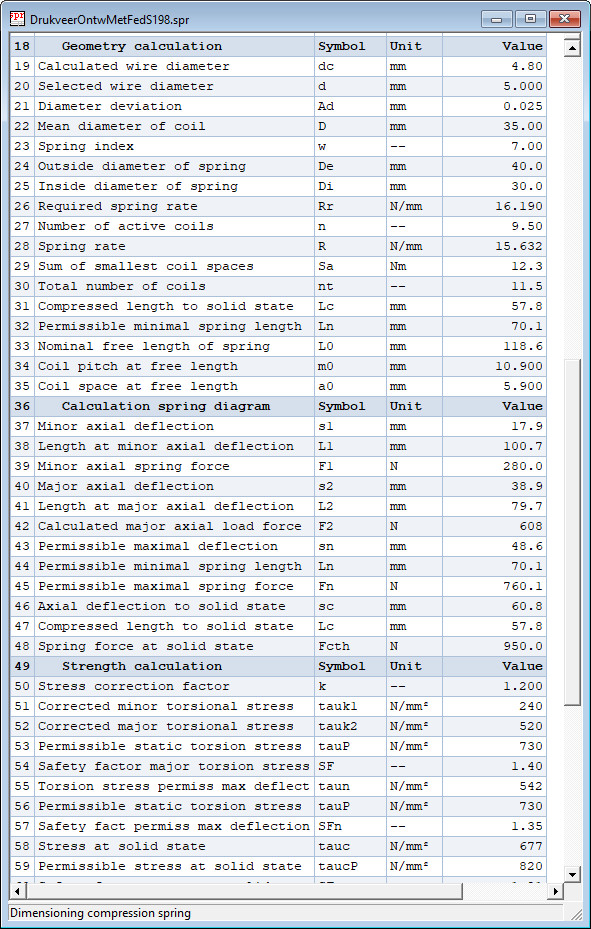### Design Compression Spring Calculation Results

The calculation results are displayed in table form on the screen, as in following example:Click here on Calculation Report Design Compression Spring to open a design calculation report in a target blank page.

## Verification Calculation of Compression Spring

Verification calculation according standard DIN EN 13096-1. The verification calculation can only be done if spring geometry and spring load is known. The safety factors on static and dynamic load on failure are calculated. The safety factor on buckling is determined. Same calculation steps as in the design operation are performed, except those for calculation of the spring dimensions. The natural frequency of the spring coils in longitudinal direction is calculated.

Click here on Calculation Report Verification Compression Spring to open a verification calculation report in a target blank page.

Go to next web page for calculation of extension springs.